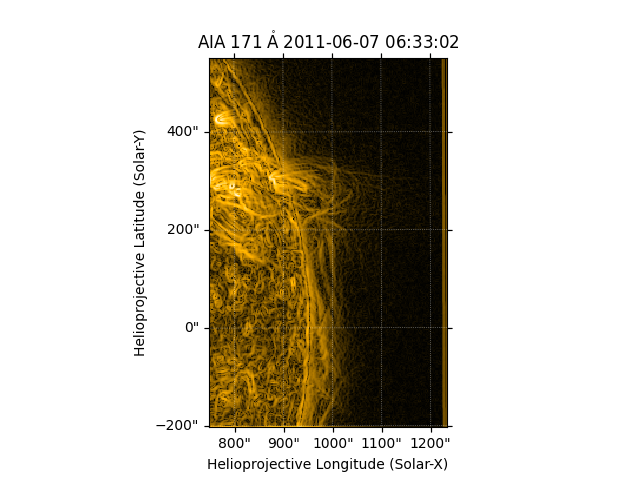# Edge Enhancing Coronal Loops¶

How to edge enhance coronal loops in an SDO/AIA image.

import matplotlib.pyplot as plt
import numpy as np
from scipy import ndimage

import astropy.units as u
from astropy.coordinates import SkyCoord

import sunpy.map
from sunpy.data.sample import AIA_171_IMAGE


We start with the sample data and crop it down to a region featuring coronal loops.

aia = sunpy.map.Map(AIA_171_IMAGE)
bottom_left = SkyCoord(750 * u.arcsec, -200 * u.arcsec, frame=aia.coordinate_frame)
top_right = SkyCoord(1500 * u.arcsec, 550 * u.arcsec, frame=aia.coordinate_frame)
aia_smap = aia.submap(bottom_left, top_right=top_right)


Next we apply an edge enhance filter to the data in both x and y directions and combine the two images together.

sx = ndimage.sobel(aia_smap.data, axis=0, mode='constant')
sy = ndimage.sobel(aia_smap.data, axis=1, mode='constant')
edge_enhanced_im = np.hypot(sx, sy)


Finally we create a new map with the edge enhanced data.

edge_map = sunpy.map.Map(edge_enhanced_im, aia_smap.meta)


Let’s plot the results.

plt.figure()
edge_map.plot()
plt.show()Total running time of the script: ( 0 minutes 0.944 seconds)

Gallery generated by Sphinx-Gallery